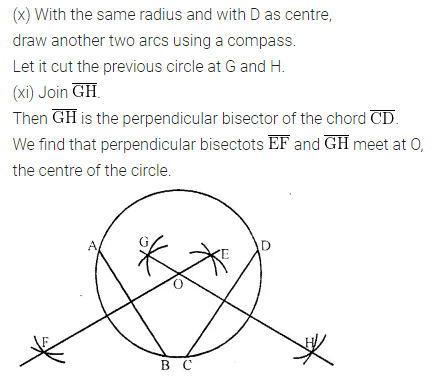ML Aggarwal Class 6 Solutions Chapter 13 Practical Geometry Ex 13.2 for ICSE Understanding Mathematics acts as the best resource during your learning and helps you score well in your exams.

## ML Aggarwal Class 6 Solutions for ICSE Maths Chapter 13 Practical Geometry Ex 13.2

Question 1.
Draw a line segment $$\overline{\mathrm{PQ}}$$ =5.6 cm. Draw a perpendicular to it from a point A outside $$\overline{\mathrm{PQ}}$$ by using ruler and compass.
Solution: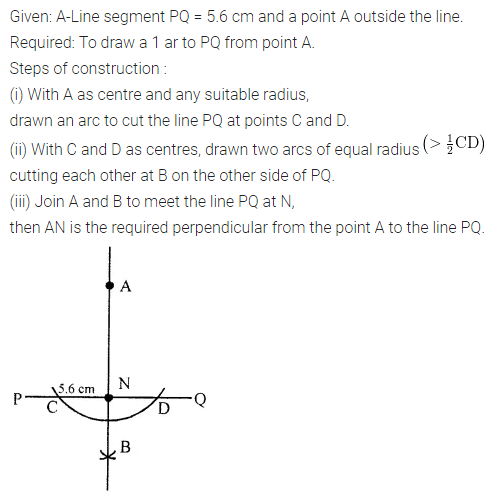Question 2.
Draw a line segment $$\overline{\mathrm{AB}}$$ = 6.2 cm. Draw a perpendicular to it at a point M on $$\overline{\mathrm{AB}}$$ by using ruler and compass.
Solution:Question 3.
Draw a line l and take a point P on it. Through P, draw a line segment $$\overline{\mathrm{PQ}}$$ perpendicular to l. Now draw a perpendicular to $$\overline{\mathrm{PQ}}$$ at Q (use ruler and compass).
Solution:Question 4.
Draw a line segment $$\overline{\mathrm{AB}}$$ of length 6.4 cm and construct its axis of symmetry (use ruler and compass).
Solution:Question 5.
Draw the perpendicular bisector of $$\overline{\mathrm{XY}}$$ whose length is 8.3 cm.
(i) Take any point P on the bisector drawn. Examine whether PX = PY.
(ii) If M is the mid-point of $$\overline{\mathrm{XY}}$$, what can you say about the lengths MX and MY?
Solution: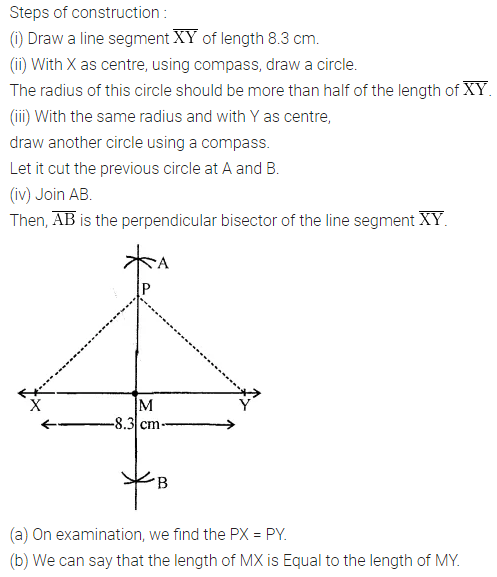Question 6.
Draw a line segment of length 8.8 cm. Using ruler and compass, divide it into four equal parts. Verify by actual measurement.
Solution: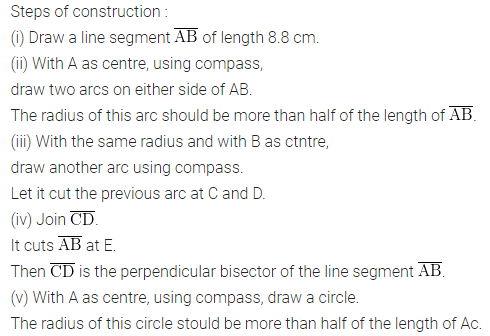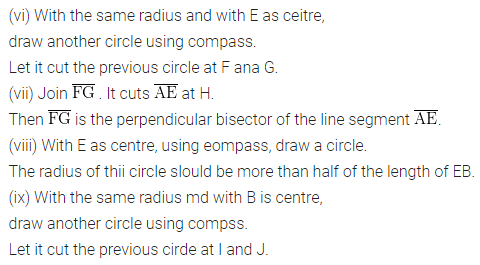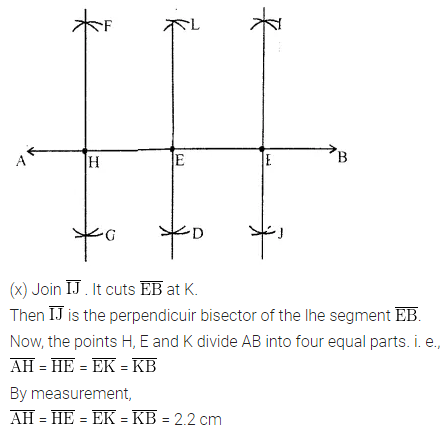Question 7.
With $$\overline{\mathrm{PQ}}$$ of length 5.6 cm as diameter, draw a circle.
Solution: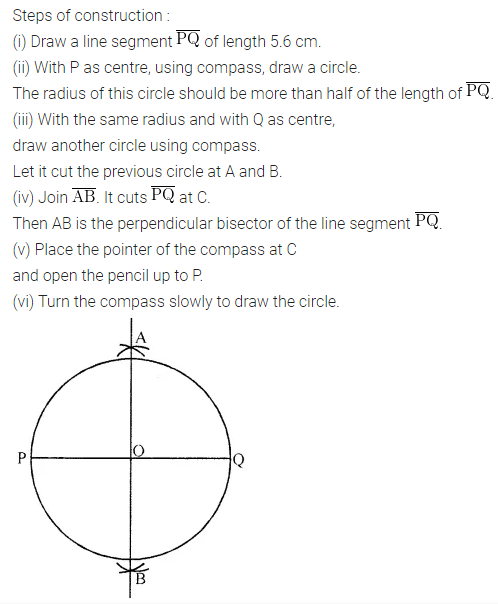Question 8.
Draw a circle with centre C and radius 4.2 cm. Draw any chord AB. Construct the perpendicular bisector of AB and examine if it passes through C.
Solution:Question 9.
Draw a circle of radius 3.5 cm. Draw any two of its (non-parallel) chords. Construct the perpendicular bisectors of these chords. Where do they meet?
Solution: